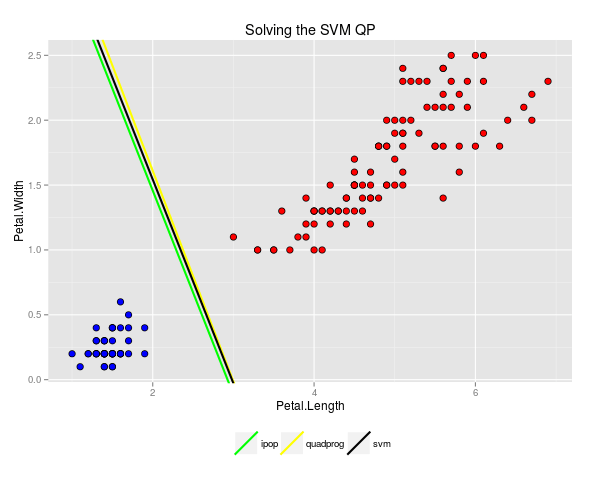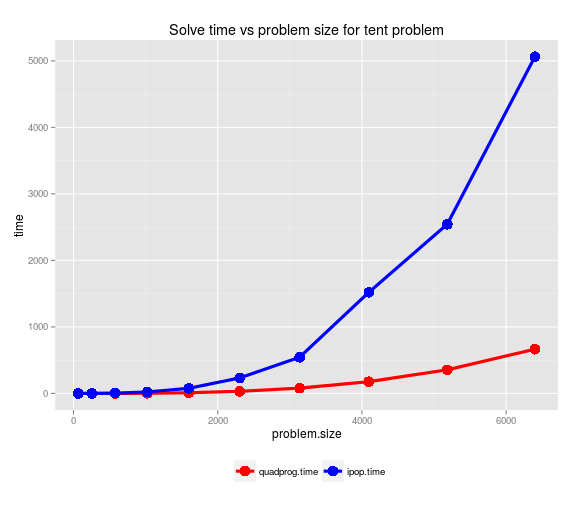# More on Quadratic Programming in R

This post is another tour of quadratic programming algorithms and applications in R. First, we look at the quadratic program that lies at the heart of support vector machine (SVM) classification. Then we’ll look at a very different quadratic programming demo problem that models the energy of a circus tent. The key difference between these two problems is that the energy minimization problem has a positive definite system matrix whereas the SVM problem has only a semi-definite one. This distinction has important implications when it comes to choosing a quadratic program solver and we’ll do some solver benchmarking to further illustrate this issue.

# QP and SVM

Let’s consider the following very simple version of the SVM problem. Suppose we have observed $y_i \in \{-1,1\}$, $X_i \in \mathbb{R}^{m}$ for $n$ (perfectly linearly separable) training cases. We let $y$ denote the $n \times 1$ vector of training labels and $X$ the $n \times m$ matrix of predictor variables. Our task is to find the hyperplane in $\mathbb{R}^m$ which “best separates” our two classes of labels $-1$ and $1$. Visually:The problem of finding this optimal hyperplane is a quadratic program (the primal SVM problem).  For computational reasons however, it is much easier to work with a related quadratic program, the SVM dual problem.  With a general kernel function, this quadratic program is:
\begin{aligned} \underset{\alpha \in \mathbb{R}^n}{\text{Maximize}}: \qquad & L\left( \alpha \right) = \sum_{i=1}^n \alpha_{i} – \frac{1}{2} \sum_{i=1}^n \sum_{j=1}^n \alpha_i \left( y_i k\left(\mathbf{X}_i, \mathbf{X}_j \right) y_j \right) \alpha_j \\ \text{Subject to:} \qquad & 0 \leq \alpha_i \leq C. \end{aligned}
In the simple case of a linear kernel, $k(\mathbf{x}, \mathbf{y}) = \mathbf{x} \cdot \mathbf{y}$ and so we can rewrite $L$ in matrix notation as
$L(\alpha) = \mathbf{1} \cdot \alpha – \alpha^T Q^TQ \alpha$
with
$Q = \left[ \begin{array}{ccc} y_1 (\mathbf{X}^T)_1 \; | & \ldots & | \; y_n (\mathbf{X}^T)_n \end{array}\right]$
where $(\mathbf{X}^T)_i$ denotes the $i$-th column of $\mathbf{X}^T$.

It is important to note that the matrix $A = Q^TQ$ is symmetric positive semi-definite since $A^T = A$ and for any nonzero $x$ in $\mathbb{R}^n$:
$x^TAx = x^TQ^T Q x = (Qx)^T (Qx) = \|Qx\|_2^2 \geq 0.$  However, in the context of SVM the matrix $A$ will usually not be positive definite.  In particular, the rank of $X^T$ is at most $m$ (number of features) which is typically much less than the number of observations $n$.  By the Rank-Nullity theorem, the nullspace of $Q$ has dimension $n-m$ which we expect to be quite large.  So intuitively quite a few non-zero $x$ map to $0$ under $Q$ and so the above inequality cannot be strengthened to a strict inequality.

The fact that the matrix $A$ is only semi-definite and not positive-definite is significant because many quadratic programming algorithms are specialized to solve only positive definite problems.  For example, R’s quadprog handles only positive definite problems, whereas solvers like kernlab’s ipop method can handle semidefinite problems.  In the specialized semidefinite case of SVM, many highly optimized algorithms exist (for example, the algorithms implemented in libsvm and liblinear).  In the following gist, we solve a separable SVM problem for Fisher’s iris data in three ways:

1. Using the e1071 wrapper around libsvm.
2. Using the semi-definite ipop solver from kernlab. Note that this general interior point solver is implemented in R and it can be quite slow when applied to larger scale problems.
3. Using quadprog’s positive definite solver with a slight perturbance to the SVM data so that the system matrix becomes positive definite. Quadprog is a wrapper around an interior point solver implemented in Fortran.

Note that only the first method is recommended for solving SVM problems in real life. The second and third methods are only included for the sake of the demonstrating the mechanics of quadratic programming.

We see that all three methods generate quite similar solutions for this highly stable and quite simple problem.# The Circus Tent Revisited

In a previous post, I explained how the solution of a quadratic program can model the shape of a circus tent.The system matrix in the circus tent problem is symmetric positive definite and therefore an ideal candidate for the quadprog solver. In the gist below, we build a series of increasingly larger circus tent problems and use this to profile the solver times of ipop and quadprog.From this simple experiment we can make the following observations:

1. For this symmetric positive definite problem, quadprog’s solver is significantly faster than ipop. This is not surprising given that quadprog is calling compiled Fortran routines whereas ipop is an R implementation.
2. The time complexity for both solvers is superlinear in the problem size. Roughly, both solvers appear to be nearly cubic in problem size.
3. Even though both the system and constraint matrices are sparse, neither solver is able to take advantage of sparse matrix representations. In a pinch, it’s worth noting that a little memory can be saved by using quadprog’s solve.QP.compact which utilizes a more compact representation of the constraint matrix $\mathrm{Amat}$.